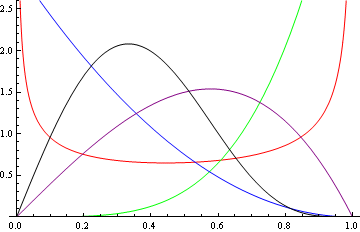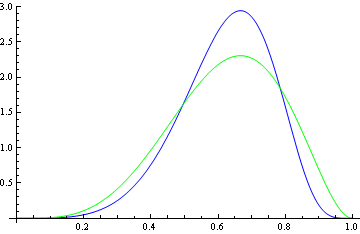# A beta-like distribution

I just stumbled across a distribution that approximates the beta distribution but is easier to work with in some ways. It’s called the Kumaraswamy distribution. Apparently it came out of hydrology. The graph below plots the density of the distribution for various parameters. If you’re familiar with the beta distribution, these curves will look very familiar.The PDF for the Kumaraswamy distribution K(a, b) is

f(x | a, b) = abxa-1(1 – xa)b-1

and the CDF is

F(x | a, b) = 1 – (1 – xa)b.

The most convenient feature of the Kumaraswamy distribution is that its CDF has a simple form. (The CDF for a beta distribution cannot be reduced to elementary functions unless its parameters are integers.)  Also, the CDF is easy to invert. That means you can generate a random sample from a K(a, b) distribution by first generating a uniform random value u and then returning

F-1(u) = (1 – (1 – u)1/b)1/a.

If you’re going to use a Kumaraswamy distribution to approximate a beta distribution, the question immediately arises of how to find parameters to get a good approximation. That is, if you have a beta(α, β) distribution that you want to approximate with a K(a, b) distribution, how do you pick a and b?

My first thought was to match moments. That is, pick a and b so that K(a, b) has the same mean and variance as beta(α, β). That may work well, but it would have to be done numerically.

Since the beta(α, β) density is proportional to xα (1-x)β-1 and the K(a, b) distribution is proportional to xa(1 – xa)b, it seems reasonable to set a = α. But how do you pick b? The modes of the two distributions have simple forms and so you could pick b to match modes:

mode K(a, b) = ((a – 1)/(ab – 1))1/a = mode beta(α, β) = (α – 1)/(α + β – 2).

Update: I experimented with the method above, and it’s OK, but not great. Here’s an example comparing a beta(1/2, 1/2) density with a K(1/2, 2 – √2) density.Here the K density matches the beta density not at the mode but at the minimum. The blue curve, the curve on top, is the beta density.

Here’s another example, this time comparing a beta(5, 3) density and a K(5, 251/40) density.Again the beta density is the blue curve, on top at the mode.

Maybe the algorithm I suggested for picking parameters is not very good, but I suspect the optimal parameters are not much better. Rather than saying that the Kumaraswamy distribution approximates the beta distribution, I’d say that the Kumaraswamy distribution is capable of assuming roughly the same shapes as the beta distribution. If the only reason you’re using a beta distribution is to get a certain density shape, the Kumaraswamy distribution would be a reasonable alternative. But if you need to approximate a beta distribution closely, it may not work well enough.

## 10 thoughts on “A beta-like distribution”

1. I got all excited about this, thinking it might simplify some computations in DCDFLIB. I worked until 2 AM on this, went to bed and came to no conclusions.

2. Gene Harris

But ever the optimist, I thought I could do better on choosing parameters. I realized around 1:30 AM it was not optimism but foolishness on my part. I thought I could do some fitting and come up with an empirical equation for estimating parameters. I was wrong!

3. Hi!

Try for your first example betaA=betaB=0.5 , K(a,b) = [4.3604471e-01 5.1398941e-01] and for the second example K(a,b) = [3.7355497e+00 3.4185949e+00]. Your page here made me work on this; the results are a couple of hours of work and hence need to be checked!

You can see my web page (software section) for other parameters, a full file of over 800 combinations.

Ponnu

4. I know this is an old post, but a related article just popped up on arxiv:

A New Generalized Kumaraswamy Distribution
Authors: Jalmar M.F. Carrasco, Silvia L.P. Ferrari, Gauss M. Cordeiro
(Submitted on 6 Apr 2010)

Abstract: A new five-parameter continuous distribution which generalizes the Kumaraswamy and the beta distributions as well as some other well-known distributions is proposed and studied. The model has as special cases new four- and three-parameter distributions on the standard unit interval. Moments, mean deviations, R’enyi’s entropy and the moments of order statistics are obtained for the new generalized Kumaraswamy distribution. The score function is given and estimation is performed by maximum likelihood. Hypothesis testing is also discussed. A data set is used to illustrate an application of the proposed distribution.

http://arxiv.org/abs/1004.0911v1

5. saba khalid khwaja

I would be obliged if anyone please let me know the truncated pdf of kumaraswamy distribution.

6. Mike Sty

This is actually pretty fascinating and useful. First-year probability students really seem to struggle with the concept of CDF, and once they see things like Beta without a closed-form CDF they can’t get their head around it.

7. Aswin Raghavan

Would gradient descent of the negative log likelihood function give MLE estimates for the parameters? The loglikehood has a nice form that can be maximized by nonlinear numerical optimizers.
log L(a,b|X)=log(a)+log(b)+\sum_i [(a-1)log x_i + (b-1)*log(1-x_i^a)]

8. Justin Winokur

I know this post is *really* old at this point, but I was just wondering if you’ve studied the affect of using a numerical integrator for the CDF of a beta (and, then a root-finder on that for inversion). I’ve used betas extensively and never found an issue nor was the computation slow by any means.

I guess there is something elegant about not needing a numerical solution, but I think that is besides the point. If you have any non-integer parameters, they were likely fit by some numerical method already!